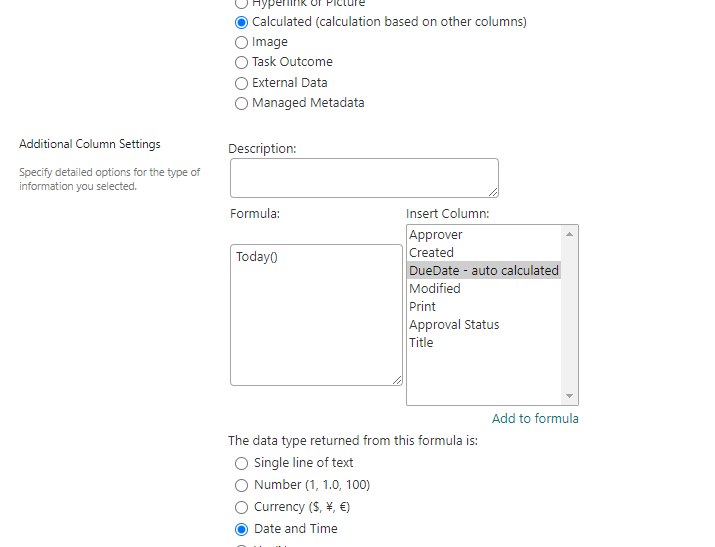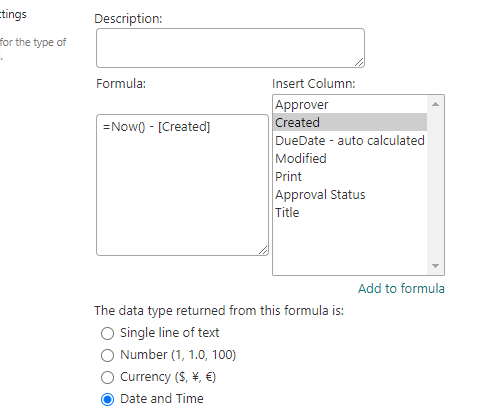# SharePoint - Use Today's Date Time in list view filter and calculated column

SharePoint provides a great way to filter views using inbuilt functions like Today() and Now(). They come very handy when you are required to get current date and time for any comparison or filtering.

These functions have been very popular throughout these years and work well with all SharePoint versions - MOSS, SharePoint 2010, SharePoint 2013, SharePoint 2016, SharePoint 2019 and SharePoint Online (Microsoft Office 365).

### ⚡️ How to use/set today's current date in calculated column --> Today()

2. Navigate to Columns section.
3. Click Create column. You may alternatively edit an existing calculated column.
4. For 'The type of information in this column is:' --> select 'Calculated (calculation based on other columns)'.
5. In Formula, use Today() for getting current date.Current date in SharePoint calculated column

⚠️ Note that calculated column values are not updated automatically and only when list item is modified. It is recommended to use Power Automate / FLOW for updating the calculated field value on regular basis.

Few examples using Today()
• Today() --> Gets current date
• Today()-Created --> Gets number of days in between Created Date and Current Date
• Today() + 1 --> Adds 1 day to Current Date
6. For 'The data type returned from this formula is: ' --> select the format for the return value, Single line of text / Number / Currency / Date and Time / Yes/No.
7. Click OK.
8. Check your column and verify the calculated value is computed correctly.

⭐ IMPORTANT - You cannot use [Today] in your formula, this will result in error "Calculated columns cannot contain volatile functions like Today and Me."

Refer this link to fix it - How to use [Today] current date time in SharePoint calculated columnCalculated columns volatile functions error

### ⚡️ How to use/set today's current date with time in calculated column --> Now()

This is similar to previous example, but more accurate up to time including minutes.
2. Navigate to Columns section.
3. Click Create column. You may alternatively edit an existing calculated column.
4. For 'The type of information in this column is:' --> select 'Calculated (calculation based on other columns)'.
5. In Formula, use Now() for getting current date and time.Current date time in SharePoint calculated column using Now

⚠️ Note that calculated column values are not updated automatically and only when list item is modified. It is recommended to use Power Automate / FLOW for updating the calculated field value on regular basis.

Few examples using Now()
• Now() --> Gets current date + time
• Now()-Created --> Gets number of days (including hours/minutes) in between Created Date and Current Date/Time
• Now() + 1 --> Adds 1 day to Current Date (including time).
6. For 'The data type returned from this formula is: ' --> select the format for the return value, Single line of text / Number / Currency / Date and Time / Yes/No.
7. Click OK.
8. Check your column and verify the calculated value is computed correctly.

### ⚡️ How to use today's current date to filter SharePoint views --> [Today]

Something which is required very frequently, filtering views to show relevant information to users. Say, displaying approval tasks which are upcoming today.
2. Navigate to Views section.
3. Open the respective view.
4. Navigate to Filter section.
5. Select 'Show items only when the following is true:'
6. For 'Show the items when column'
• Select the column from the drop down
• Select the condition which should evaluate to true
• Enter the formula to calculate using [Today]
7. Click OK.
8. Verify the view is filtered as expected.
9. Few examples using [Today]
• DueDate = [Today]
• DueDate <= [Today] AND Resolved=No
• ReminderDate = [Today]-2
• Created = [Today]-5
The combinations would vary based on your needs, you can even combine multiple conditions using And/Or. Use the correct one to filter the list items.

This does not stop here. Though SharePoint provides you powerful ready functions like [Today] and [Me], you will have to put in extra efforts to fulfill the actual requirement - like adding logic to skip weekends. In many cases, excel formulas may also work with SharePoint calculated fields.

⛏️ Tip - For calculated column fields, the value will not auto update unless the list item is modified. This has been a pain for developers and admins. There could be multiple possibilities to update the field value, choose the best fit for you -

1. Power Automate / FLOW to update item (preferred)
2. PowerShell script
3. Azure Functions
4. Logic Apps